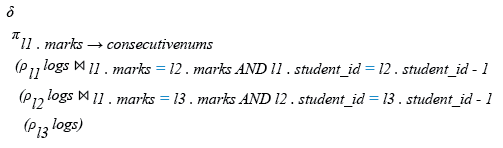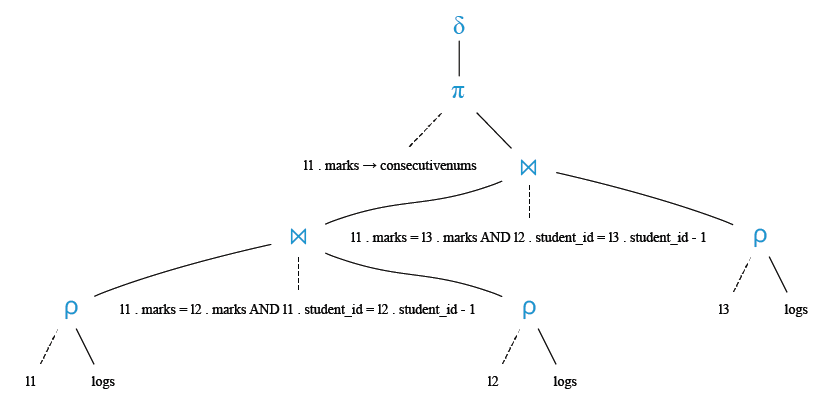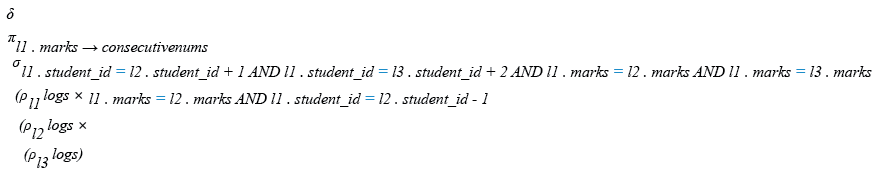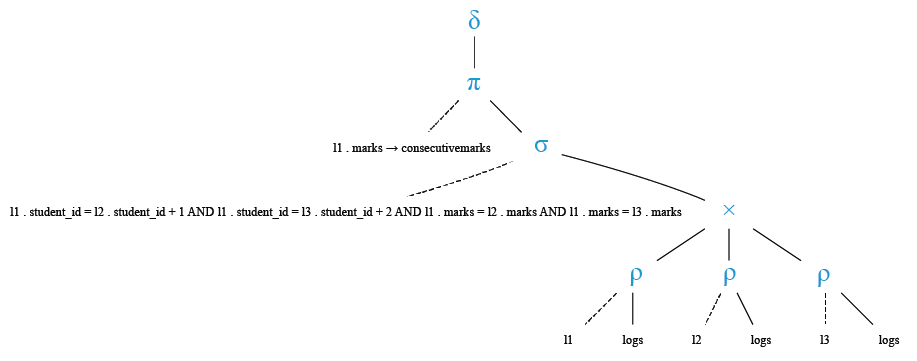﻿ SQL Challenges-1: Consecutive Numbers - w3resource# SQL Challenges-1: Consecutive Numbers

## SQL Challenges-1: Exercise-4 with Solution

From the following table, write a SQL query to find the marks, which appear at least thrice one after another without interruption. Return the number.

Input:

Table: logs

Structure:

FieldTypeNullKeyDefaultExtra
student_idint(11)YES
marksint(11)YES

Data:

student_idmarks
10183
10279
10383
10483
10583
10679
10779
10883

Sample Solution:

SQL Code(MySQL):

``````CREATE TABLE IF NOT EXISTS logs (student_id int, marks int);
TRUNCATE TABLE logs;
INSERT INTO logs (student_id, marks) VALUES ('101', '83');
INSERT INTO logs (student_id, marks) VALUES ('102', '79');
INSERT INTO logs (student_id, marks) VALUES ('103', '83');
INSERT INTO logs (student_id, marks) VALUES ('104', '83');
INSERT INTO logs (student_id, marks) VALUES ('105', '83');
INSERT INTO logs (student_id, marks) VALUES ('106', '79');
INSERT INTO logs (student_id, marks) VALUES ('107', '79');
INSERT INTO logs (student_id, marks) VALUES ('108', '83');
select * from logs;
SELECT DISTINCT L1.marks AS  ConsecutiveNums
FROM (logs L1 JOIN logs L2 ON L1.marks = L2.marks AND L1.student_id = L2.student_id-1)
JOIN logs L3 ON L1.marks = L3.marks AND L2.student_id = L3.student_id-1;
```
```

Sample Output:

```ConsecutiveNums|
---------------|
83|
```

Relational Algebra Expression:Relational Algebra Tree:Relational Algebra Expression:Relational Algebra Tree:Solution-1:

``````SELECT DISTINCT L1.marks AS  ConsecutiveNums
FROM (logs L1 JOIN logs L2 ON L1.marks = L2.marks AND L1.student_id = L2.student_id-1)
JOIN logs L3 ON L1.marks = L3.marks AND L2.student_id = L3.student_id-1;
``````

Solution-2:

``````SELECT DISTINCT L1.marks AS ConsecutiveMarks
FROM logs AS L1, logs AS L2, logs AS L3
WHERE L1.student_id = L2.student_id +1
AND L1.student_id = L3.student_id +2
AND L1.marks = L2.marks AND L1.marks = L3.marks;
``````

SQL Code Editor:

Previous: Nth Highest Sale amount.
Next: Duplicate Emails.

﻿

## SQL: Tips of the Day

How to select the nth row in a SQL database table?

Basically, PostgreSQL and MySQL supports the non-standard:

```SELECT...
LIMIT y OFFSET x
```

Oracle, DB2 and MSSQL supports the standard windowing functions:

```SELECT * FROM (
SELECT
ROW_NUMBER() OVER (ORDER BY key ASC) AS rownumber,
columns
FROM tablename
) AS foo
WHERE rownumber <= n
```

Database: SQL

Ref: https://bit.ly/3zPxcD8